### Categorical Data Encoding

• date 29th January, 2020 |
• by Prwatech |

# Approaches for Categorical Data Encoding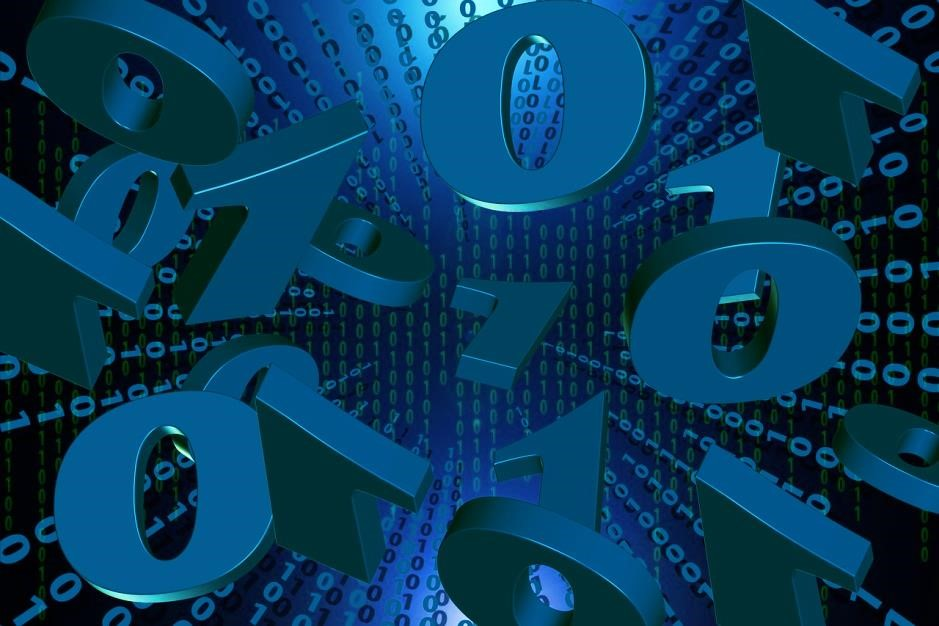Categorical Data Encoding, Are you the one who is looking forward to knowing the types of categorical data encoding in machine learning? Or the one who is looking forward to knowing Categorical Data Encoding concepts in Machine Learning or Are you dreaming to become to certified Pro Machine Learning Engineer or Data Scientist, then stop just dreaming, get your Data Science certification course from India’s Leading Data Science training institute.

Many Machine Learning algorithms need to covert categorical data into numerical data as they cannot handle categorical variables. Also, the performance of algorithms will vary based on how the categorical variables are encoded. In this blog, we will learn types of categorical data encoding and how to convert categorical data to numerical data with detailed information. Do you want to know categorical data encoding in machine learning, So follow the below mentioned Python categorical data encoding guide from Prwatech and take advanced Data Science training like a pro from today itself under 10+ Years of hands-on experienced Professionals.

In practical datasets, there is a variety of categorical variables. These variables represent different characteristics which are generally stored in text values. For example: In some cases, the gender category like Male, Female, weather can have categories like ‘sunny’, ‘rainy’ or ‘cold’, size having categories like ‘Small’, ‘Medium’, ‘Large’. We have to utilize this kind of data for analysis. Many machine learning algorithms can process these categorical values without further manipulation or encoding, but there are many algorithms too that do not support these formats. So in those cases, it is mandatory that, the data set should be manipulated to get these text attributes into numerical values for further processing.

## Types of Categorical Data Encoding

There cannot be a single answer about how to approach this problem. Different approaches come with different potential impact on the result of the analysis. So here an overview is given for data encoding approaches used mostly for categorical data.

### Label Encoding in Machine Learning

It is one of the popular techniques used to encode categorical data. It is nothing but converting each text value in a column to a number. Label encoding means to convert the labels into some machine-readable values format. For example, if the column named ‘Intensity’ contains categorical values like LOW, MEDIUM, HIGH. Then with label encoding, a unique number is assigned to each one alphabetically as.

HIGH=0

LOW =1

MEDIUM =2

From here we come to know the weightage of a particular category.

### How to convert Categorical Data to Numerical Data on Label Encoding?

Let’s see an example on Label Encoding:

 age Gender BMI Diabetes region 19 female 27.9 yes southwest 18 male 33.77 no southeast 28 male 33 no southeast 33 male 22.705 yes northwest 32 male 28.88 no northwest 31 female 25.74 no southeast 46 female 33.44 yes southeast 37 female 27.74 no northwest 37 male 29.83 no northeast 60 female 25.84 no northwest

Let’s go step by step:

### First, we have to check the columns, which contain dtype as a category. For that:

df

Output: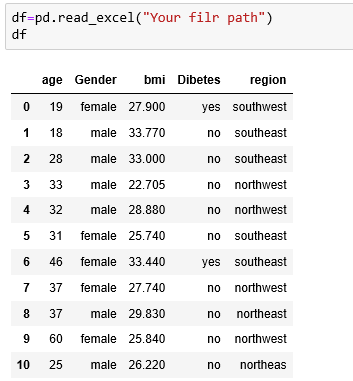### Then check the data types of columns in df

df.dtypes

Output: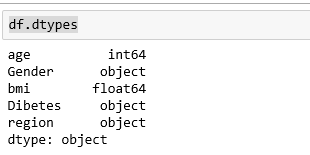### It shows that although columns ‘Gender’, ’Diabetes’ and ‘region’ are of categorical type, their data type is ‘object’. Let’s test on the field ‘Gender’. We will convert its type to category.

df[‘Gender’]=df[‘Gender’].astype(‘category’)

df.[‘Gender’].dtype

Output: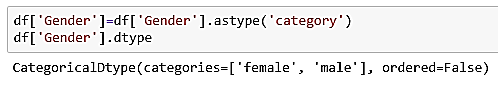### Now we can apply codes on the column named ‘Gender’ to convert its text values into numerical values.

df[‘Gender’]=df[‘Gender’].cat.codes

df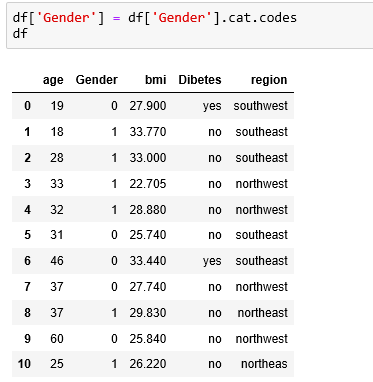As we can see in the result the column has been converted into 0 and 1 (female – 0, male – 1). In this manner, we can apply the cat.codes to all columns.

In the above case, the columns were limited and we could count the categories in each column easily. But the solution gets critical if the number of columns and categories gets increased. So in that case scikit-learn’s Label Encoder comes in picture.

For this, we have to import LabelEncoder from sklearn.preprocessing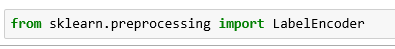And now we can select and encode the expected columns as follows: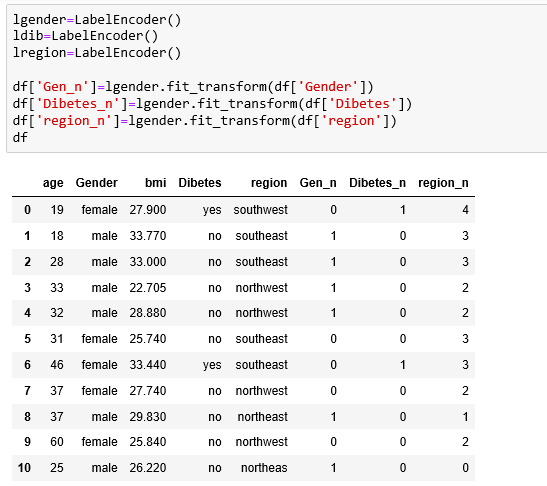Here we get all selected columns in the form of numerical values.

But in the case of Label encoding, there is a problem of misinterpretation of numerical values while analysis and processing. For example, here region category is present, in that different regions are assigned with different numeric values. In further processing, the more weightage can be given to the category ‘southwest’ as it has value 4. This unwanted weight interpretation can miss guiding the machine learning algorithm.

### One Hot Encoding in Machine Learning:

One hot encoding means a strategy to encode each category value into a new column and allocate a 1 or 0 (True/False) value to the column. Unlike Label Encoding, it will not weight values improperly. Let’s see the above example with one hot encoder. For that, we have to import Label Encoder as shown in the above example.  Then we will apply function get_dummies as follows:

df=pd.get_dummies(df,columns=[‘Gender’,‘Dibetes’,‘region’])

df

The result will be dummied columns with categories in those, for selected columns: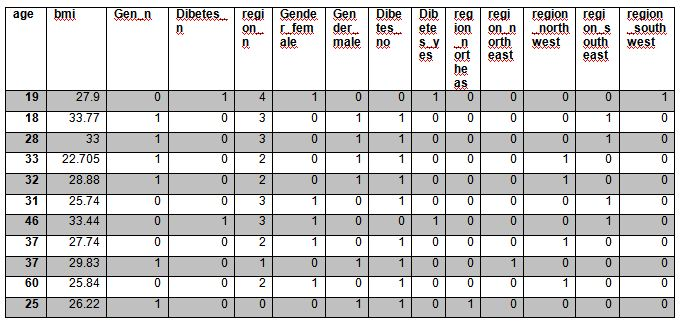Although one-hot encoding solves the problem of unequal weights given to categories, it is not very useful when there are too many categories featuring a column, since that will result in the creation of new columns in the table leading to an increase in the total dataset.

### Binary Encoding in Machine Learning

In this technique, first, the categories are encoded in integers, and then those integers are converted into binary code, then the digits from that binary string are split into separated columns. In comparison with one hot encoder, this technique encodes data with lower dimensionality.  We can use a binary encoder by just installing category_encoders as follows:

pip install category_encoders

import category_encoders as ce

Now we can apply binary encoder as follows:

dfbin=df.copy()

binencode=ce.BinaryEncoder(cols=[‘Gender’, ‘Dibetes’,‘region’])

df_binary =binencode.fit_transform(dfbin)

df_binary

### Result: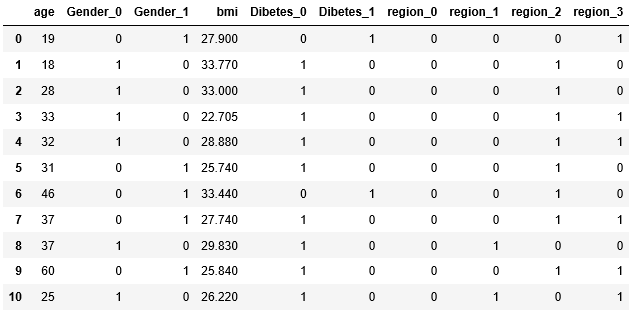We hope you understand Categorical Data Encoding in Machine Learning concepts and types of categorical data encoding, how to convert categorical data to numerical data. Get success in your career as a Data Scientist by being a part of the Prwatech, India’s leading Data Science training institute in Bangalore.

### Quick Support